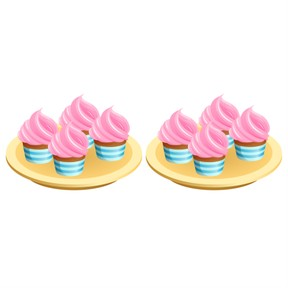Solving the 8 times table

# Solving the 8 times table

I can solve times 8 multiplication facts.8,000 schools use Gynzy92,000 teachers use Gynzy1,600,000 students use Gynzy

## General

Students will learn multiplication strategies for the times 8 facts. Students will apply the skills of halving and doubling to solve times 8 facts. Instruction and practice will cover skip counting and multiples along with using memory tricks, such as rhymes and chants.

## Standards

CCSS.Math.Content.3.OA.B.5

## Learning objective

Students will be able to solve times 8 multiplication facts.

## Introduction

Students will practice halving and doubling numbers using mental math. This is practice for a strategy they will use with the times 8 facts.

## Instruction

Students will work with the strategy of halving and doubling and then discuss if they find this a useful approach. Next, students will practice skip counting by 8. They will be shown the pattern of these multiples and then practice writing them and using them to solve the facts. Instruction will follow on the use of rhymes and chants such as “Two eights fell on the floor, when you picked them up, they were 64!” Students then solve facts and match answers using “Couch Potatoes.” Students will also apply strategies they’ve learned to solve word problems. In the “What Did You Learn” section, they will demonstrate knowledge of facts using a timed set of flashcards.

## Quiz

Students respond to ten multiple-choice and true/false questions.

## Closing

Students will divide into teams and take turns identifying math problems as a “Fact” or “Fib.” They will score correct answers to declare a champion.

### The online teaching platform for interactive whiteboards and displays in schools

• Save time building lessons

• Manage the classroom more efficiently

• Increase student engagement